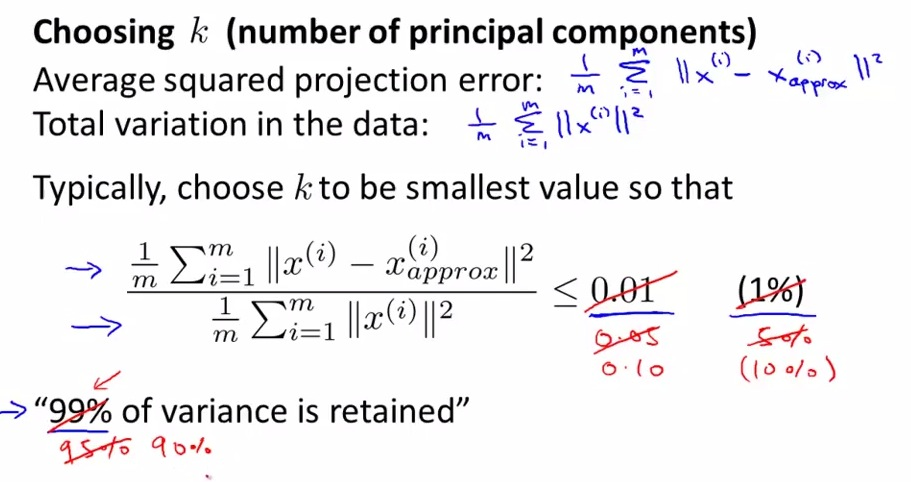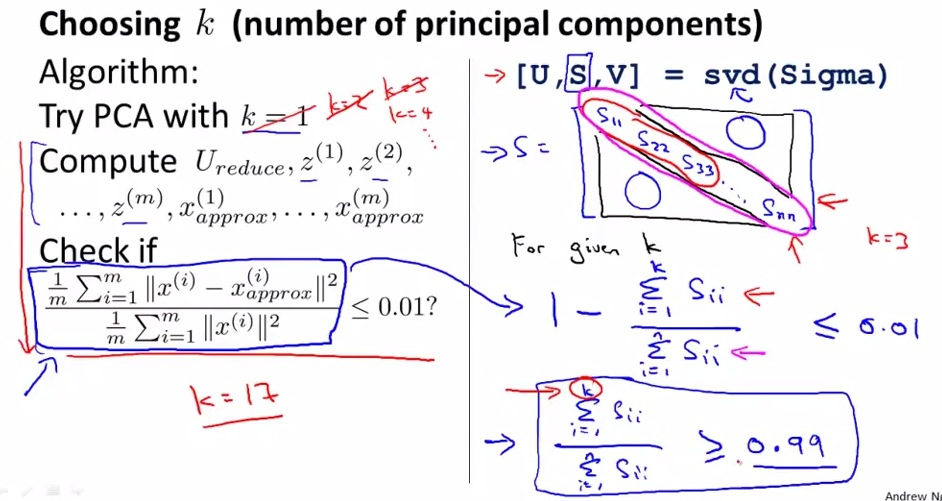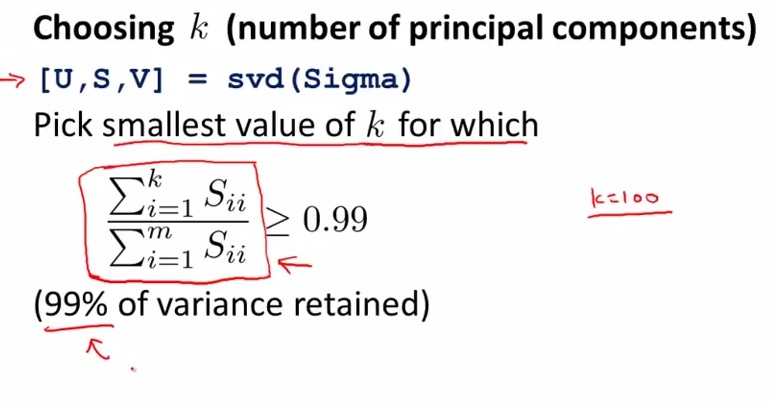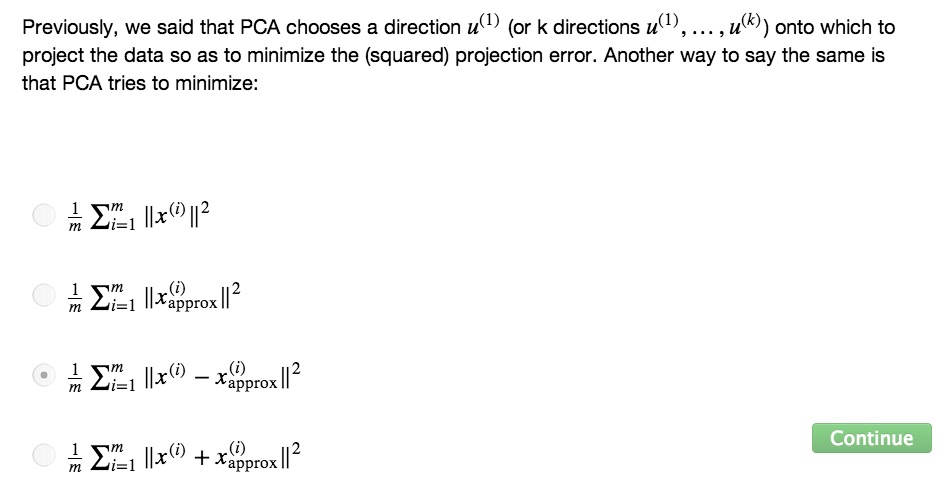• n dimensional features in large data scale usually have arround thousand number that most of them are highly correlated
• This lesson will show how to reduce for example thousand features(n) to hundred features(k) effectively while still retain most of the data• For choosing k, we at least have to take some measurement, in value, how many % we retain the original data
• Usually we set about 99%, 95%, 90% . The sentence that preferred to use is "% of variance is retained".
• Lot more simpler than saying that "we choose k unit, because..... and .... and the result of error is ........"
• typical common use is >= 95% data
• so % retained is Average squared projection error/Total variation in the data• Check to see if k = 1 is satisfied the requirement. If it don't then increase k by one every iteration
• Really inefficient if we use the computation for every iteration i
• matrix S in USV is  diagonal matrix (other zero) that we can compute every iteration(much more simple)
• Remember that k in PCA is a quantity value of the data that retained, and based on k, how much % variance that was retained, in other words, how much % of the data that retained based on the original
• Alternatively, we can init k = 1;
• Then for k, Compute like the graph on the right,  choose the smallest value so that "99% variance retained" satisfied• If we try to manually set k, then try to provide some back-up by formula that given above.
• That way others see your recommendation and approve it.
• PCA try to minimize the error for the projection line between original data (x) and data projection (x.approx)• SUMMARY
• thousand dimensional data and highly correlated, using PCA will reduce/compressed data by very large vector while retain most of the data